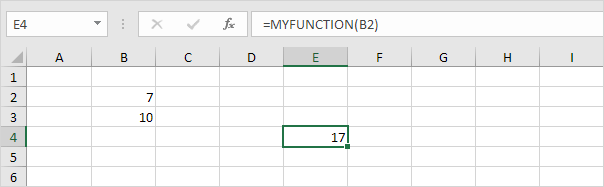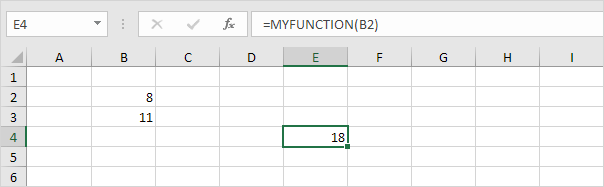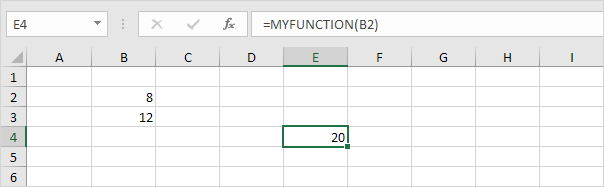# Volatile Functions

By default, UDF's (User Defined Functions) in Excel VBA are not volatile. They are only recalculated when any of the function's arguments change. A volatile function will be recalculated whenever calculation occurs in any cells on the worksheet. Let's take a look at an easy example to explain this a bit more.

1. Open the Visual Basic Editor and click Insert, Module.

Create a function called MYFUNCTION which returns the sum of the selected cell and the cell below this cell.

2. Add the following code lines:

Function MYFUNCTION(cell As Range)

MYFUNCTION = cell.Value + cell.Offset(1, 0).Value

End Function

3. Now you can use this function, just like any other Excel function.4. This is a non-volatile function. Non-volatile functions are only recalculated when any of the function's arguments change. Change the value of cell B2 to 8.5. Now change the value of cell B3 to 11.Explanation: the non-volatile function is not recalculated when any other cell on the sheet changes.

6. Update the function as follows to make the function volatile:

Function MYFUNCTION(cell As Range)

Application.Volatile
MYFUNCTION = cell.Value + cell.Offset(1, 0).Value

End Function

7. Change the value of cell B3 to 12.

Result:Note: you need to enter the function again to make it volatile (or refresh it by placing your cursor in the formula bar and pressing enter).

Go to Next Chapter: Application Object